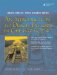# Parameter Passing by Value

By default, C++ parameters are passed by value. When a function is called, a temporary (local) copy of each argument object is made and placed on the program stack. Only the local copy is manipulated inside the function, and the argument objects from the calling block are not affected by these manipulations. The temporary stack variables are all destroyed when the function returns. A useful way to think of value parameters is this: Value parameters are merely local variables that are initialized by copies of the corresponding argument objects that are specified when the function is called. Example 5.11 gives a short demonstration.

Example 5.11. src/functions/summit.cpp

 ```#include int sumit(int num) { int sum = 0; for (; num ; --num) <-- 1 sum += num; return sum; } int main() { using namespace std; int n = 10; cout << n << endl; cout << sumit(n) << endl; cout << n << endl; <-- 2 return 0; } Output: 10 55 10 ```   (1)The parameter gets reduced to 0. (2)See what sumit() did to n.

If a pointer is passed to a function, a temporary copy of that pointer is placed on the stack. Changes to that pointer will have no effect on the pointer in the calling block. For example, the temporary pointer could be assigned a different value (see Example 5.12).

Example 5.12. src/functions/pointerparam.cpp

 ```#include using namespace std; void messAround(int* ptr) { *ptr = 34; <-- 1 ptr = 0; <-- 2 } int main() { int n(12); <-- 3 int* pn(&n); <-- 4 cout << "n = " << n << " pn = " << pn << endl; messAround(pn); <-- 5 cout << "n = " << n << " pn = " << pn << endl; return 0; } Output: n = 12 pn = 0xbffff524 n = 34 pn = 0xbffff524 ```   (1)Change the value that is pointed to. (2)Change the address stored by ptr. (3)Initialize an int. (4)Initialize a pointer that points to n. (5)See what is changed by messAround().

In the output we display the hexidecimal value of the pointer pn as well as the value of n so that there can be no doubt about what was changed by the action of the function.

### Parameter Passing by ReferenceAn Introduction to Design Patterns in C++ with Qt 4
ISBN: 0131879057
EAN: 2147483647
Year: 2004
Pages: 268